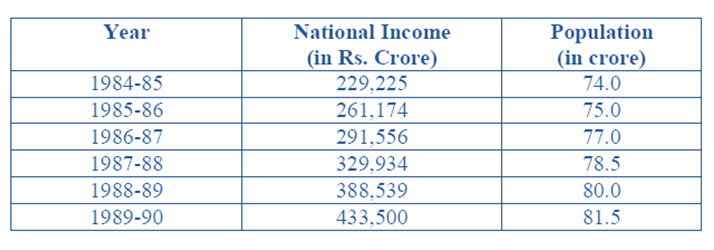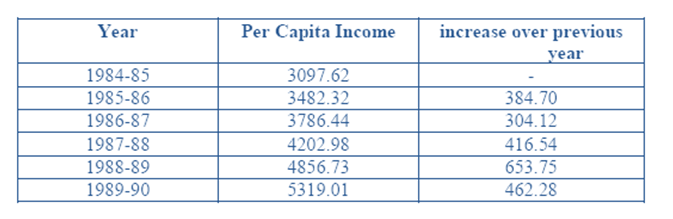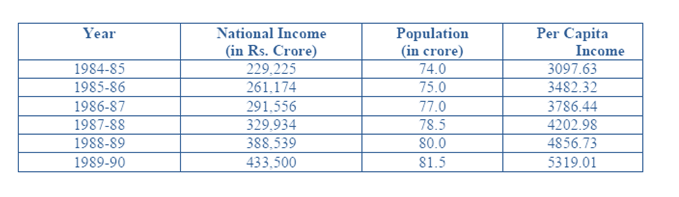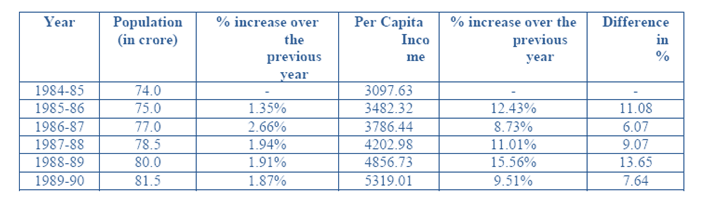The following table gives the national income and the population of a country for the years 1984 – 85to 1989 – 90. For each of the following questions choose the best alternative:Question 1: The increase in the per capita income compared to the previous year is lowest for the year:
(a) 1985-86
(b) 1986-87
(c) 1987-88
(d) 1989-90

Question 2: The per capita income is highest for the year:
(a) 1984-85
(b) 1985-86
(c) 1987-88
(d) 1989-90

Question 3: The difference between the percentage increase in per capita income and the percentage increase in the population compared to the previous year is highest for the year:
(a) 1985-86
(b) 1986-87
(c) 1987-88
(d) 1988-89

Question 4: The rate of increase in population was lowest in the year:
(a) 1985-86
(b) 1987-88
(c) 1989-90
(d) None of these

Question 5: Increase in the per capita income compared to the previous year among the years given below was highest for theyear:
(a) 1985-86
(b) 1986-87
(c) 1987-88
(d) 1989-90

We can make the following calculation: Per Capita Income= (National Income) / (Population)

The table highlights the calculated values:The increase is lowest for the year 1986 – 87 = Rs. 304 .12

We can make the following calculation: Per Capita Income=(National Income) / (Population)

The table highlights the calculated values:We can see from the above table that the Per Capita Income is highest for the year 1989 – 90 = (433,500/81.5)= Rs. 5319

We extend the above table and make the following calculations:Hence it is highest for the year 1988-89 viz.13.65